# Basic Calculation Skills

?

735 + 280 = 1015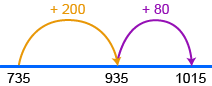827 + 564 = 1391         800 + 500 = 1300     20 + 60 = 80     7 + 4 = 11   1391

Add numbers matching place values in columns. If the answer to any column is 10 or bigger, carry the 10s to the next column.

7 2   Units: 3 + 1 + 2 = 6   2 5 1   Tens: 6 + 5 + 7 = 18 (8 carry 1) + 14 6 3   Hundreds: 4 + 1 + 2 = 7   7 8 6

1 of 14

## Subtract in columns

Here are two methods for subtracting numbers using place-value columns.

Trade 10 from the next place value.

9 6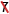15 – 6  4  8   3  2  7

2 of 14

## Multiplication Strategies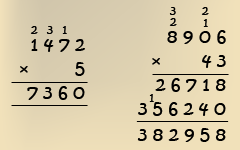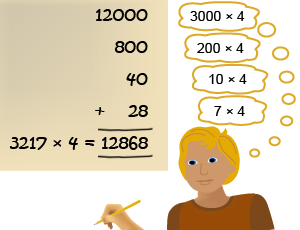3 of 14

## Division Strategies

72 ÷ 4     OR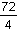OR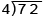4 of 14

## Remainders

Remainders can be written in different ways.

Imagine sharing 7 cupcakes between 2 people.Each person would receive three cakes with one leftover.

7 ÷ 2 = 3 r 1Or each person gets three and a half cakes.

7 ÷ 2 = 3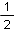or 3.5

5 of 14

## Mixed Multiplication and Division

You can change the order when multiplying and dividing.

Sometimes rearranging the problem can make working easier.

24 × 37 ÷ 8 = 24 ÷ 8 × 37   = 3 × 37   = 117

6 of 14

Integers are numbers which are not a fraction.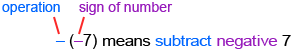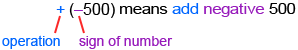## Rules

Below is a summary of the meaning of pairs of signs.

Two like signs become +
– (–9) = + 9
+ (+9) = + 9

Two different signs become
+ (–9) = – 9
– (+9) = – 9

##### EXAMPLE

Evaluate these three calculations.

20 + (–7)
20 – (–7)
20 – (+7)

##### SOLUTION

20 + (–7) = 20 – 7   = 13 20 – (–7) = 20 + 7   = 27 20 – (+7) = 20 – 7   = 13

7 of 14

## Opposite numbers

Opposite numbers have the same size but are on opposite sides of zero.

So 3 and –3 are opposites.

The sum of a number and its opposite is always zero.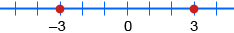Since –3 and +3 are opposites:
+3 + (–3) = 0
–3 + (+3) = 0

8 of 14

## Rules for multiplying or dividing

Two LIKE signs give a positive answer:
( + ) × ( + ) = ( + )
( − ) × ( − ) = ( + )
( + ) ÷ ( + ) = ( + )
( − ) ÷ ( − ) = ( + )
Two DIFFERENT signs give a negative answer:
( + ) × ( − ) = ( − )
( − ) × ( + ) = ( − )
( + ) ÷ ( − ) = ( − )
( − ) ÷ ( + ) = ( − )

##### Examples

−12 × −2 = 24

9 × −7 = −63

−6 ÷ −2 = 3

−44 ÷ 11 = −4

Note: Positive numbers are written without their + sign.

9 of 14

## Powers and Directed Numbers

Powers are a special form of expressing multiplication.

(–3)2 = –3 × –3 = 9

(–2)3 = –2 × –2 × –2 = –8

10 of 14

## Order Of Operations

B I D M A S

Brackets, Indices, Divison, Multiplication, Addition, Subtraction

11 of 14

## Inverse Operations

The four operations are related to each other. Operations are inverses of each other if one undoes the effect of the other.

• Adding is the inverse of subtracting.
• Multiplying is the inverse of dividing.
• Taking a square root is the inverse of squaring a number.
• Taking the cube root is the inverse of cubing a number.

You can use inverse operations to check answers to calculations.

When a calculation involves more than one operation, you have to reverse the order of the inverse operations to check the answer.

12 of 14

## Fraction Lines

Fraction lines also act as grouping symbols. The line shows the numerator as one group and the denominator as another.

##### EXAMPLE

Evaluate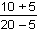.

##### SOLUTION=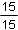=1   The fraction bar works like grouping symbols: calculate the top and bottom separately, and then divide.

13 of 14

## Operations With Square Roots

Square root signs are similar to brackets. Do any calculations within square root signs before taking the square root.

##### EXAMPLE

Find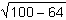.

##### SOLUTION

Do the subtraction within the square root sign first.=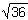= 6

Note:≠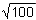–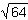(–= 10 – 8 = 2)

14 of 14